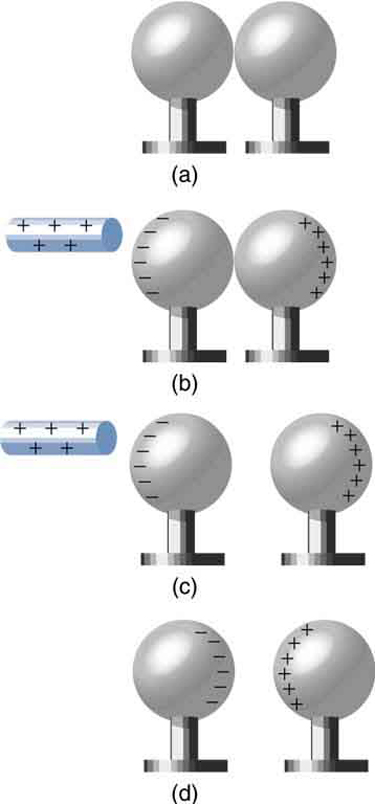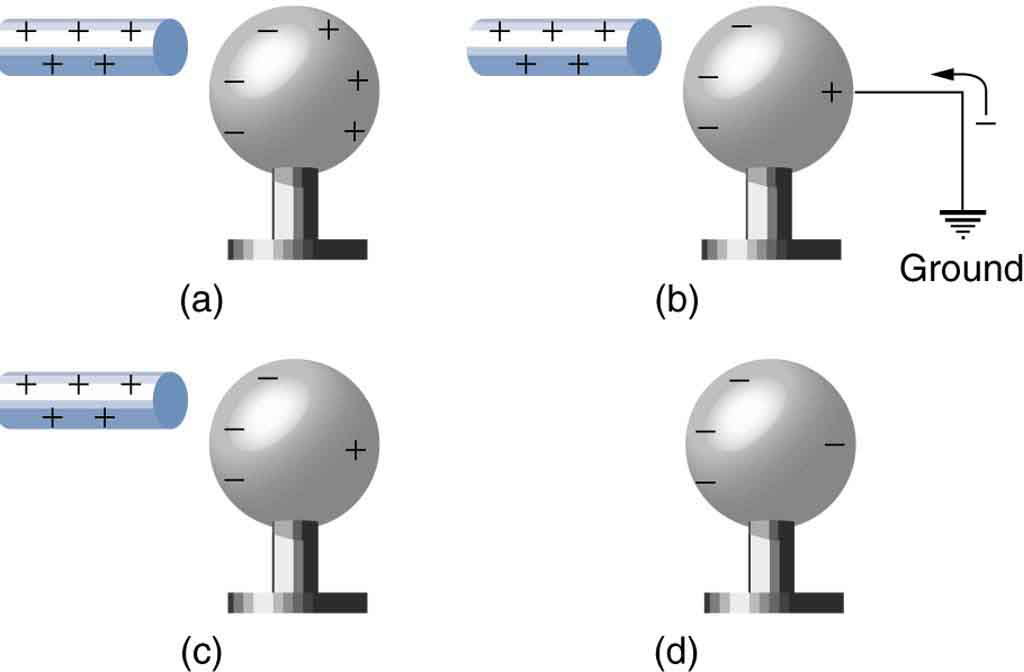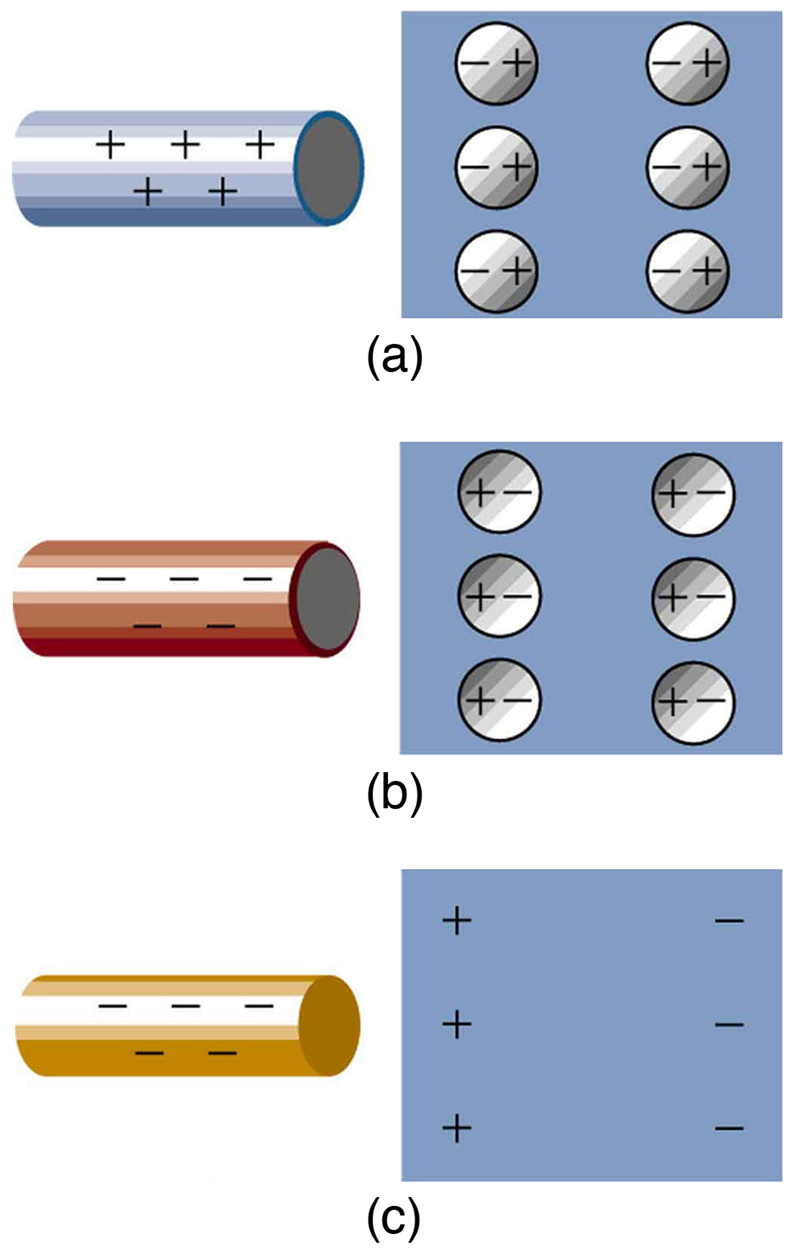# 18.2 Conductors and insulators  (Page 2/10)

 Page 2 / 10

Electrostatic repulsion in the leaves of the charged electroscope separates them. The electrostatic force has a horizontal component that results in the leaves moving apart as well as a vertical component that is balanced by the gravitational force. Similarly, the electroscope can be negatively charged by contact with a negatively charged object.

## Charging by induction

It is not necessary to transfer excess charge directly to an object in order to charge it. [link] shows a method of induction    wherein a charge is created in a nearby object, without direct contact. Here we see two neutral metal spheres in contact with one another but insulated from the rest of the world. A positively charged rod is brought near one of them, attracting negative charge to that side, leaving the other sphere positively charged.

This is an example of induced polarization    of neutral objects. Polarization is the separation of charges in an object that remains neutral. If the spheres are now separated (before the rod is pulled away), each sphere will have a net charge. Note that the object closest to the charged rod receives an opposite charge when charged by induction. Note also that no charge is removed from the charged rod, so that this process can be repeated without depleting the supply of excess charge.

Another method of charging by induction is shown in [link] . The neutral metal sphere is polarized when a charged rod is brought near it. The sphere is then grounded, meaning that a conducting wire is run from the sphere to the ground. Since the earth is large and most ground is a good conductor, it can supply or accept excess charge easily. In this case, electrons are attracted to the sphere through a wire called the ground wire, because it supplies a conducting path to the ground. The ground connection is broken before the charged rod is removed, leaving the sphere with an excess charge opposite to that of the rod. Again, an opposite charge is achieved when charging by induction and the charged rod loses none of its excess charge.Charging by induction. (a) Two uncharged or neutral metal spheres are in contact with each other but insulated from the rest of the world. (b) A positively charged glass rod is brought near the sphere on the left, attracting negative charge and leaving the other sphere positively charged. (c) The spheres are separated before the rod is removed, thus separating negative and positive charge. (d) The spheres retain net charges after the inducing rod is removed—without ever having been touched by a charged object.Charging by induction, using a ground connection. (a) A positively charged rod is brought near a neutral metal sphere, polarizing it. (b) The sphere is grounded, allowing electrons to be attracted from the earth’s ample supply. (c) The ground connection is broken. (d) The positive rod is removed, leaving the sphere with an induced negative charge.Both positive and negative objects attract a neutral object by polarizing its molecules. (a) A positive object brought near a neutral insulator polarizes its molecules. There is a slight shift in the distribution of the electrons orbiting the molecule, with unlike charges being brought nearer and like charges moved away. Since the electrostatic force decreases with distance, there is a net attraction. (b) A negative object produces the opposite polarization, but again attracts the neutral object. (c) The same effect occurs for a conductor; since the unlike charges are closer, there is a net attraction.

what is physics
what are the basic of physics
faith
tree physical properties of heat
tree is a type of organism that grows very tall and have a wood trunk and branches with leaves... how is that related to heat? what did you smoke man?
what are the uses of dimensional analysis
Dimensional Analysis. The study of relationships between physical quantities with the help of their dimensions and units of measurements is called dimensional analysis. We use dimensional analysis in order to convert a unit from one form to another.
Emmanuel
meaning of OE and making of the subscript nc
Negash
kinetic functional force
what is a principal wave?
A wave the movement of particles on rest position transferring energy from one place to another
Gabche
not wave. i need to know principal wave or waves.
Haider
principle wave is a superposition of wave when two or more waves meet at a point , whose amplitude is the algebraic sum of the amplitude of the waves
kindly define principal wave not principle wave (principle of super position) if u can understand my question
Haider
what is a model?
hi
Muhanned
why are electros emitted only when the frequency of the incident radiation is greater than a certain value
b/c u have to know that for emission of electron need specific amount of energy which are gain by electron for emission . if incident rays have that amount of energy electron can be emitted, otherwise no way.
Nazir
Nazir
what is ohm's law
states that electric current in a given metallic conductor is directly proportional to the potential difference applied between its end, provided that the temperature of the conductor and other physical factors such as length and cross-sectional area remains constant. mathematically V=IR
ANIEFIOK
hi
Gundala
A body travelling at a velocity of 30ms^-1 in a straight line is brought to rest by application of brakes. if it covers a distance of 100m during this period, find the retardation.
just use v^2-u^2=2as
Gundala
how often does electrolyte emits?
alhassan
just use +€^3.7°√π%-4¢•∆¥%
v^2-u^2=2as v=0,u=30,s=100 -30^2=2a*100 -900=200a a=-900/200 a=-4.5m/s^2
akinyemi
what's acceleration
The change in position of an object with respect to time
Mfizi
Acceleration is velocity all over time
Pamilerin
hi
Stephen
It's not It's the change of velocity relative to time
Laura
Velocity is the change of position relative to time
Laura
acceleration it is the rate of change in velocity with time
Stephen
acceleration is change in velocity per rate of time
Noara
what is ohm's law
Stephen
Ohm's law is related to resistance by which volatge is the multiplication of current and resistance ( U=RI)
Laura
acceleration is the rate of change. of displacement with time.
the rate of change of velocity is called acceleration
Asma
how i don understand
how do I access the Multiple Choice Questions? the button never works and the essay one doesn't either
How do you determine the magnitude of force
mass × acceleration OR Work done ÷ distance
SeemaByByBy OpenStaxBy Nicole BartelsBy Richley CrapoBy Dan ArielyBy Saylor FoundationBy Michael SagBy Mary CohenBy Dravida Mahadeo-J...By Madison ChristianBy OpenStax